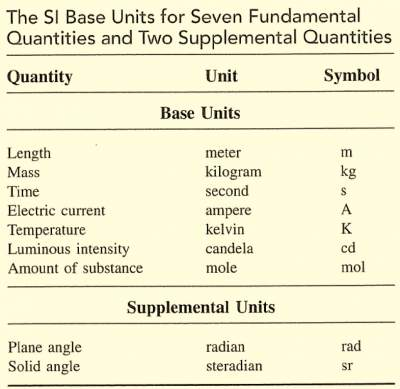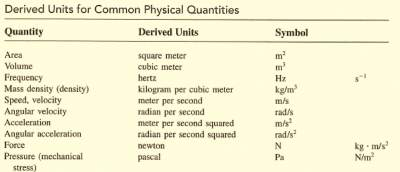# Introduction to Measurements and Basic Units

## Introduction

All engineers must use the same language in their communications, and one of these universal communication tools is the units of measurements.
Physical quantities such as length, weight, time, speed, force, and mass are measured with standard units.
Therefore the magnitude of a physical quantity is given by a number and standard unit of measurement. Examples: 3 meters, 10 inches, 7 kilograms, 5 ounces.
SI units are the international system of units (system international) as illustrated below:
The rendered complex table example is shown here:• Note that the above units are stated in MKS (Meter-Kilogram-Second) also known as SI Units.
• Another French standard unite of measurement is called the CGS (Centimeter-Gram-Second).
• Both MKS (Meter-Kilogram-Second) and CGS (Centimeter-Gram-Second) are metric units, but the MKS units are more commonly used worldwide.

### Derived Units

Many quantities can be measured including volume, pressure, speed, and force that are combinations of two or more fundamental quantities.
Combinations of these quantities are referred to as derived quantities and they are measured in derived units as depicted below:
The rendered table example is shown here:### Examples

1. Convert 5 kg to mg. Answer: 5000 g
2. Convert 750 m to km. Answer: 0.75 km
3. The density of Alumunium is 2.7 g/cm3. What is the density in kilograms per cubic meter? Answer: 2,700
4. Find the speed of 90 km/hr in meters per second. Answer: 25

For more details, please contact me here.
Date of last modification: Summer , 2019